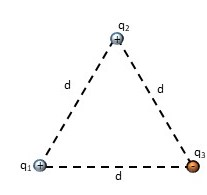# The point charges in the figure have the following values: q_1= +1.8 \mu C, q_2= +6.5 \mu C, q_3=...

## Question:

The point charges in the figure have the following values: {eq}q_1= +1.8 \mu C, q_2= +6.5 \mu C, q_3= -0.89 \mu {/eq} (let the +x-axis pont to the right).

Given that the distance d= 4.35 cm, find the magnitude and direction of the electrostatic force exerted on the point charge {eq}q_1. {/eq}## Coulombic Force Between Two Charges

According to Coulomb's law, the force of attraction or repulsion between two charges is given by,

{eq}\vec F=\frac{kq_1q_2}{r^2}\hat r {/eq}

Where,

• {eq}k=8.99\times10^9 {/eq}
• {eq}q_1,q_2 {/eq} are the charges,
• {eq}r {/eq} is the separation between the charges and
• {eq}\hat r {/eq} is the unit vector from source charge to the test charge where we measure the force.

Become a Study.com member to unlock this answer! Create your account

Given:

{eq}q_1=1.8\times10^{-6}\ C\\ q_2=6.5\times10^{-6}\ C\\ q_3=-0.89\times10^{-6}\ C\\ d=4.35\times10^{-2}\ m {/eq}

Let us assume that the...Coulomb's Law: Variables Affecting the Force Between Two Charged Particles

from

Chapter 6 / Lesson 3
39K

In the 18th century, Charles Coulomb uncovered the secrets of electrostatic force between charged particles. The results of his experiments led to what is now known as Coulomb's Law, which tells us how force, charge, and distance are all related.